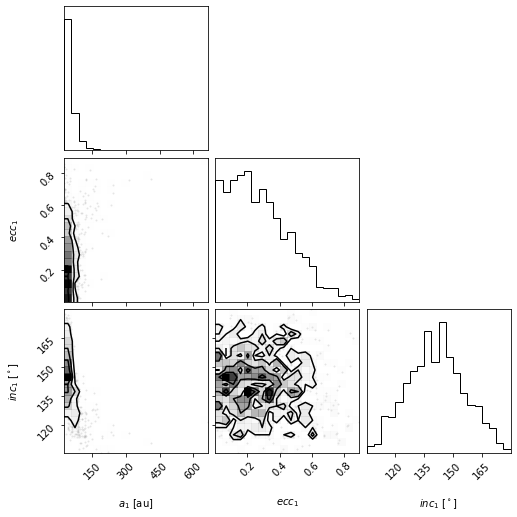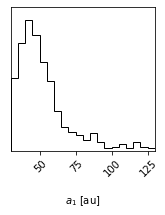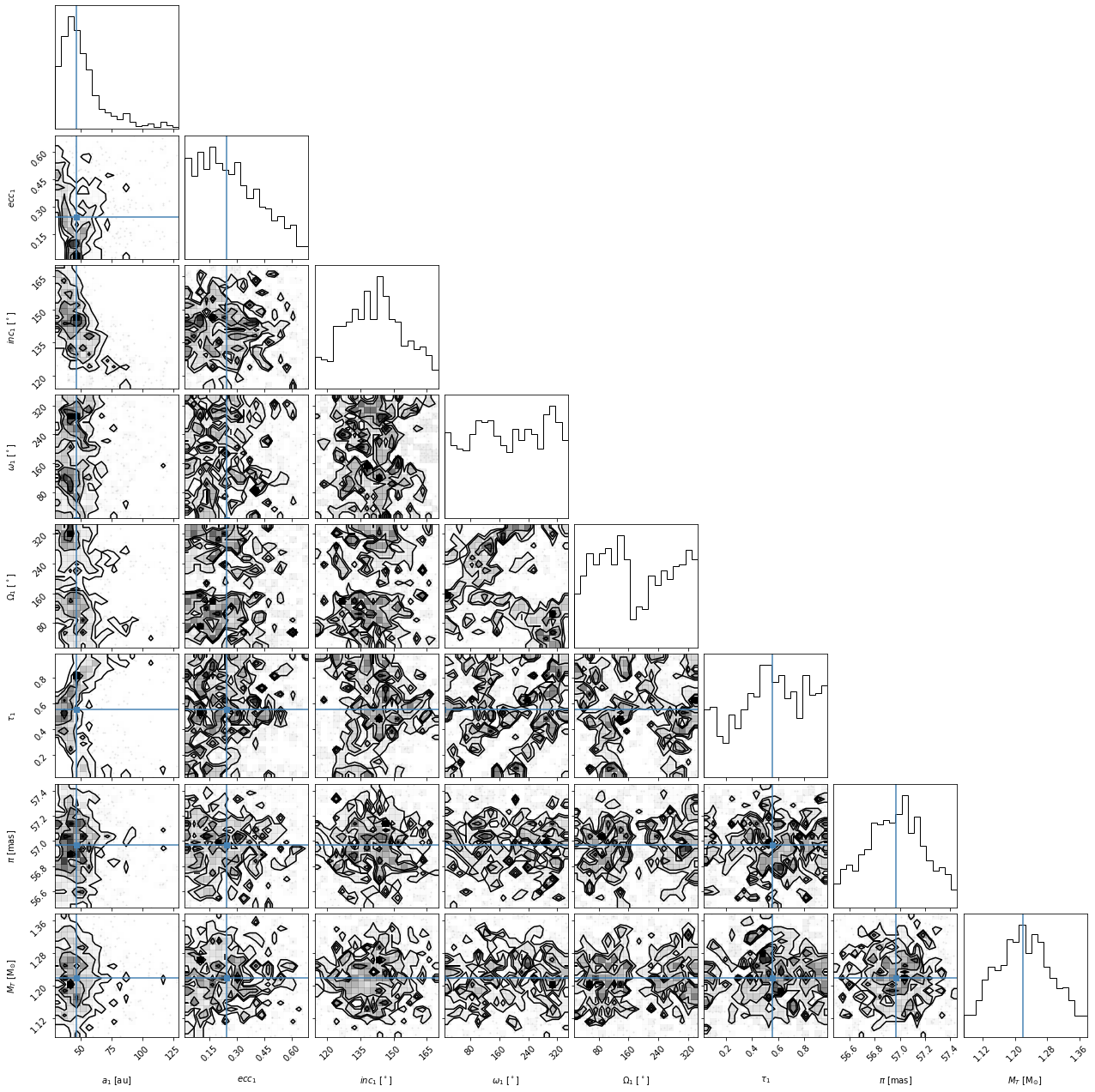by Henry Ngo (2018)

The results.py module contains several plotting functions to visualize the results of your orbitize orbit fit. Basic uses of these functions are covered in the OFTI and MCMC tutorials. Here, we will examine these plotting functions more deeply. This tutorial will be updated as more features are added to orbitize.

## 1. Test orbit generation with OFTI¶

In order to have sample data for this tutorial, we will use OFTI to generate some orbits for a published dataset on the GJ 504 system. The following code block is from the OFTI Tutorial , with 10000 orbits generated. Please see that tutorial for details.

:

import orbitize.driver

myDriver = orbitize.driver.Driver(
'{}/GJ504.csv'.format(orbitize.DATADIR), # relative or absolute path to data file
'OFTI', # name of algorithm for orbit-fitting
1, # number of secondary bodies in system
1.22, # total system mass [M_sun]
56.95, # parallax of system [mas]
mass_err=0.08, # mass error [M_sun]
plx_err=0.26 # parallax error [mas]
)
s = myDriver.sampler
orbits = s.run_sampler(10000)

WARNING: KEPLER: Unable to import C-based Kepler's equation solver. Falling back to the slower NumPy implementation.
10000/10000 orbits found


## 2. Accessing a Results object with computed orbits¶

After computing your orbits from either OFTI or MCMC, they are accessible as a Results object for further analysis and plotting. This object is an attribute of s, the sampler object defined above.

:

myResults = s.results # array of MxN array of orbital parameters (M orbits with N parameters per orbit)


It is also useful to save this Results object to a file if we want to load up the same data later without re-computing the orbits.

:

myResults.save_results('plotting_tutorial_GJ504_results.hdf5')


:

myResults?


Note that you can also add more computed orbits to a results object with myResults.add_samples():

:

myResults.add_samples?


## 3. (Optional) Load up saved results object¶

If you are skipping the generation of all orbits because you would rather load from a file that saved the Results object generated above, then execute this block to load it up. Otherwise, skip this block (however, nothing bad will happen if you run it even if you generated orbits above).

:

import orbitize.results
if 'myResults' in locals():
del myResults # delete existing Results object
myResults = orbitize.results.Results() # create empty Results object


## 4. Using our Results object to make plots¶

There are currently two plotting functions available: plot_corner() makes a corner plot and plot_orbits() displays some or all of the computed orbits. Both plotting functions return matplotlib.pyplot.figure objects, which can be displayed, further manipulated with matplotlib.pyplot functions, and saved.

:

%matplotlib inline
import matplotlib.pyplot as plt


### 4.1 Corner plots¶

This function is a wrapper for corner.py and creates a display of the 2-D covariances between each pair of parameters as well as histograms for each parameter. These plots are known as “corner plots”, “pairs plots”, and “scatterplot matrices”, as well as other names.

:

corner_figure = myResults.plot_corner()#### Choosing which parameters to plot¶

Sometimes, the full plot with all parameters is not what we want. Let’s use the param_list keyword argument to plot only semimajor axis, eccentricity and inclination. Here are the possible string labels you can enter for param_list and the corresponding orbit fit parameter:

Label

Parameter name

sma1

semimajor axis

ecc1

eccentricity

inc1

inclination

aop1

argument of periastron

pan1

position angle of nodes (aka longitude of ascending node)

tau1

epoch of periastron passage (expressed as a fraction of orbital period past a specified offset)

mtot

system mass

plx

system parallax

Note: for labels with numbers, the number corresponds to the companion (sma1 is the first object’s semimajor axis, sma2 would be the second object, etc)

:

corner_figure_aei = myResults.plot_corner(param_list=['sma1','ecc1','inc1'])#### Limiting which samples to display¶

By picking out the panels we show, the plot can be easier to read. But in this case, we see that the plotted x-range on semimajor axis does show the posterior very well. This function will pass on all corner.corner() keywords as well. For example, we can use corner’s range keyword argument to limit the panels to only display 95% of the samples to avoid showing the long tails in the distribution.

:

corner_figure_aei_95 = myResults.plot_corner(param_list=['sma1','ecc1','inc1'], range=(0.95,0.95,0.95))For other keywords you can pass to corner, see the corner.py API.

#### Making single variable histogram plots¶

One use of the param_list keyword is to just plot the histogram for the distribution of one single orbit fit parameter. We can do this by just providing one single parameter.

:

histogram_figure_sma1 = myResults.plot_corner(param_list=['sma1'], range=(0.95,))#### Axis label customization¶

The axis labels seen on the above plots are the default labels that orbitize passes to corner.py to make these plots. We can override these defaults by passing our own labels through the labels keyword parameter as per the corner.py API.

Note that the length of the list of labels should match the number of parameters plotted.

:

# Corner plot with alternate labels
corner_figure_aei_95_labels = myResults.plot_corner(
param_list=['sma1','ecc1','inc1'],
range=(0.95,0.95,0.95),
labels=('SMA (AU)', 'eccen.', 'inc. (deg)')
)#### Overplotting best fit (“truth”) values¶

One feature of corner.py is to overplot the contours and histograms with a so-called “truth” value, which we can use for many purposes. For example, if we are sampling from a known distribution, we can use it to plot the true value to compare with our samples. Or, we can use it to mark the location of the mean or median of the distribution (the histogram and contours make it easy to pick out the most likely value or peaks of the distribution but maybe not the mean or median). Here is an example of overplotting the median on top of the distribution for the full corner plot.

:

import numpy as np
median_values = np.median(myResults.post,axis=0) # Compute median of each parameter
range_values = np.ones_like(median_values)*0.95 # Plot only 95% range for each parameter
corner_figure_median_95 = myResults.plot_corner(
range=range_values,
truths=median_values
)Overall, we find that some of the parameters have converged well but others are not well constrained by our fit. As mentioned above, the output of the plot_corner() methods are matplotlib Figure objects. So, if we wanted to save this figure, we might use the savefig() method.

:

corner_figure_median_95.savefig('plotting_tutorial_corner_figure_example.png')


### 4.2 Orbit Plot¶

The plot_orbits method in the Results module allows us to visualize the orbits sampled by orbitize!. The default call to plot_orbits will draw 100 orbits randomly chosen out of the total orbits sampled (set by parameter num_orbits_to_plot). In addition, to draw each of these orbits, by default, we will sample each orbit at 100 evenly spaced points in time throughout the orbit’s orbital period (set by parameter num_epochs_to_plot). These points are then connected by coloured line segments corresponding to the date where the object would be at that point in the orbit. By default, orbits are plotted starting in the year 2000 (set by parameter start_mjd) and are drawn for one complete orbital period. We usually choose to begin plotting orbits at the first data epoch, using this keyword as illustrated below.

:

epochs = myDriver.system.data_table['epoch']

orbit_figure = myResults.plot_orbits(
start_mjd=epochs # Minimum MJD for colorbar (here we choose first data epoch)
)
orbit_figure.savefig('example_orbit_figure.png',dpi=250)#### Customizing orbit plot appearence¶

In the above figure, we see that the x and y axes are RA and Dec offsets from the primary star in milliarcseconds. By default the axes aspect ratio is square, but we can turn that off. This is normally not recommended but for some situations, it may be desirable.

(Note: Each call to plot_orbits selects a different random subset of orbits to plot, so the orbits shown in the figures here are not exactly the same each time).

:

orbit_figure_non_square = myResults.plot_orbits(
start_mjd=epochs,
square_plot=False
)The colorbar shows how the line segment colors correspond to the date, beginning at the first data epoch. We can also turn off the colourbar.

:

orbit_figure_no_colorbar = myResults.plot_orbits(
start_mjd=epochs,
show_colorbar=False
)We can also modify the color and ending-epoch of the separation/position angle panels as follows:

:

orbit_figure_no_colorbar = myResults.plot_orbits(
start_mjd=epochs,
sep_pa_color='lightblue',
sep_pa_end_year = 2100.0
)#### Choosing how orbits are plotted¶

Plotting one hundred orbits may be too dense. We can set the number of orbits displayed to any other value.

:

orbit_figure_plot10 = myResults.plot_orbits(
start_mjd=epochs,
num_orbits_to_plot=10
)We can also adjust how well we sample each of the orbits. By default, 100 evenly-spaced points are computed per orbit, beginning at start_mjd and ending one orbital period later. Decreasing the sampling could lead to faster plot generation but if it is too low, it might not correctly sample the orbit, as shown below.

:

orbit_figure_epochs10 = myResults.plot_orbits(
start_mjd=epochs,
num_epochs_to_plot=10,
num_orbits_to_plot=10
)In this example, there is only one companion in orbit around the primary. When there are more than one, plot_orbits will plot the orbits of the first companion by default and we would use the object_to_plot argument to choose a different object (where 1 is the first companion).

### 4.3 Working with matplotlib Figure objects¶

The idea of the Results plotting functions is to create some basic plots and to return the matplotlib Figure object so that we can do whatever else we may want to customize the figure. We should consult the matplotlib API for all the details. Here, we will outline a few common tasks.

Let’s increase the font sizes for all of the text (maybe for a poster or oral presentation) using matplotlib.pyplot. This (and other edits to the rcParams defaults) should be done before creating any figure objects.

:

plt.rcParams.update({'font.size': 16})


Now, we will start with creating a figure with only 5 orbits plotted, for simplicity, with the name orb_fig. This Figure object has two axes, one for the orbit plot and one for the colorbar. We can use the .axes property to get a list of axes. Here, we’ve named the two axes ax_orb and ax_cbar. With these three objects (orb_fig and ax_orb, and ax_cbar) we can modify all aspects of our figure.

:

orb_fig = myResults.plot_orbits(start_mjd=epochs, num_orbits_to_plot=5)
ax_orb, ax_cbar, ax_sep, ax_pa  = orb_fig.axesFirst, let’s try to add a figure title. We have two options. We can use the Figure’s suptitle method to add a title that spans the entire figure (including the colorbar).

:

orb_fig.suptitle('5 orbits from GJ-504 fits') # Adds a title spanning the figure
orb_fig

:Alternatively, we can just add the title over the Ra/Dec axes instead.

:

orb_fig.suptitle('') # Clears previous title
ax_orb.set_title('5 orbits from GJ-504 fits') # sets title over Axes object only
orb_fig

:We can also change the label of the axes, now using matplotlib.Axes methods.

:

ax_orb.set_xlabel('$\Delta$(Right Ascension, mas)')
ax_orb.set_ylabel('$\Delta$(Declination, mas)')
orb_fig

:If we want to modify the colorbar axis, we need to access the ax_cbar object

ax_orb.set_xlabel(‘$$\Delta$$RA [mas]’) ax_orb.set_ylabel(‘$$\Delta$$Dec [mas]’) # go back to what we had before ax_cbar.set_title(‘Year’) # Put a title on the colorbar orb_fig

We may want to add to the plot itself. Here’s an exmaple of putting an star-shaped point at the location of our primary star.

:

ax_orb.plot(0,0,marker="*",color='black',markersize=10)
orb_fig

:Here’s another example of adding datapoints with error bars to the separation/position angle panels:

:

from astropy.time import Time

# grab data from Driver object
data_tab = myDriver.system.data_table
print(data_tab)

epochs_yr = Time(data_tab['epoch'], format='mjd').decimalyear
sep = data_tab['quant1']; sep_err = data_tab['quant1_err']
pa = data_tab['quant2']; pa_err = data_tab['quant2_err']

# add data to sep panel
ax_sep.errorbar(
epochs_yr, sep, sep_err,
color='purple', linestyle='', fmt='o', zorder=3
)

# add data to PA panel
ax_pa.errorbar(
epochs_yr, pa, pa_err,
color='purple', linestyle='', fmt='o', zorder=3
)

# zoom in a bit
ax_sep.set_xlim(2011.25,2013)
ax_pa.set_xlim(2011.25,2013)
ax_sep.set_ylim(2450,2550)
ax_pa.set_ylim(323,330)

orb_fig

    epoch      object quant1 quant1_err quant2 quant2_err quant_type
-------------- ------ ------ ---------- ------ ---------- ----------
55645.95      1 2479.0       16.0 327.94       0.39      seppa
55702.89      1 2483.0        8.0 327.45       0.19      seppa
55785.015      1 2481.0       33.0 326.84       0.94      seppa
55787.935      1 2448.0       24.0 325.82       0.66      seppa
55985.19400184      1 2483.0       15.0 326.46       0.36      seppa
56029.11400323      1 2487.0        8.0 326.54       0.18      seppa
56072.30200459      1 2499.0       26.0 326.14       0.61      seppa

:And finally, we can save the figure objects.

:

orb_fig.savefig('plotting_tutorial_plot_orbit_example.png')